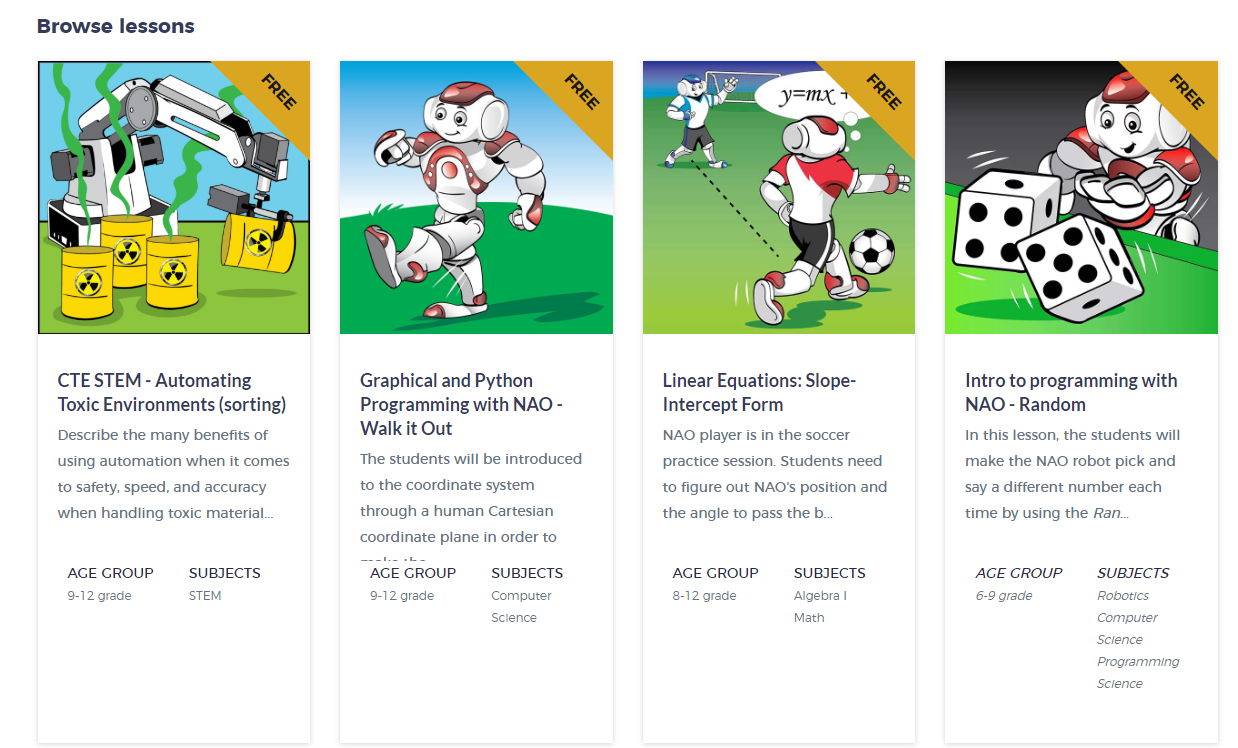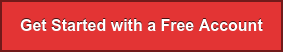<img alt="" src="https://secure.smart-enterprise-52.com/266730.png" style="display:none;">

## RobotLAB Blog

### Everything You Need To Know About Robotics in Education and Businesses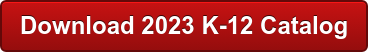This is a new educational channel called Smart Friday!

Every Friday we will share with you an informational piece about a topic we care about! You can use it in your classroom, share it, learn and enjoy it!.

Today, we are going to learn about the Triangle Inequality Theorem. Let's get started!In Mathematics, the triangle inequality states that for any triangle, the sum of the lengths of any two sides must be greater than the length of the remaining side.A + B > C B + C > A A + C > B

* Remember that this rule must be satisfied for all 3 conditions of the sides.10 + 3 > 410 + 4 > 34 + 3 > 10*As soon as 1 pair do not sum up to a greater lenght than the third, you know that the 3 sides cannot make a triangle

Let's see how much you have learned!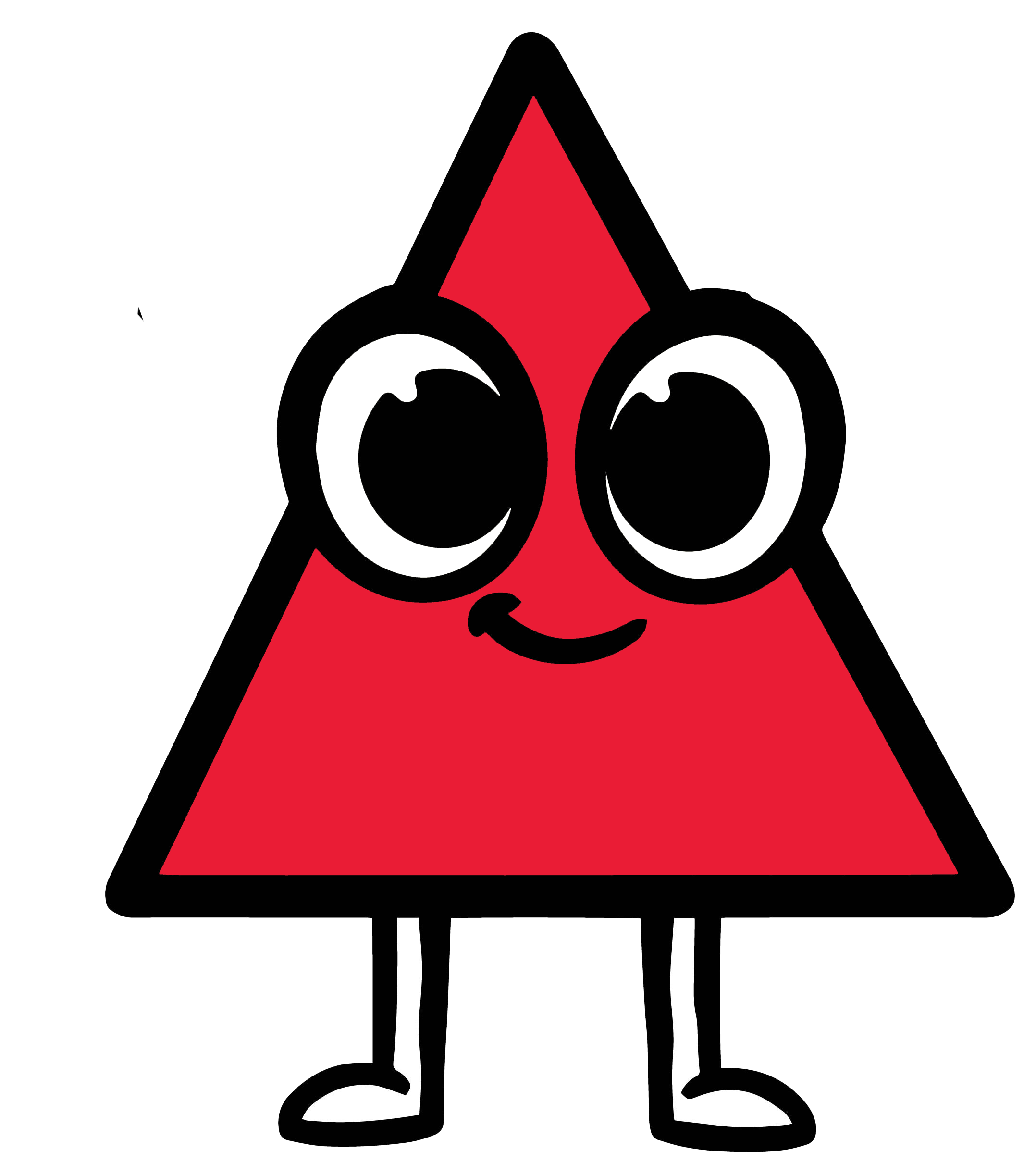Answer the following problems applying the Triangle Inequality Theorem!

Problem 1

If A=7  and C=8  what would be the value of B to complete the theorem?a: 15

b: 8

c: 20

If you chose Congratulations! that is the right answer because if you sum 8+8 =16 and  that is higher than 7 and higher than 15. Remember that the theorem says that the sum of two sides can't be less or equal to the third side that's why A and C are not correct!

Problem 2

If  B= 5  and C= 15 the sum of these two sides is 20 what is the number that represents A to solving the theorem?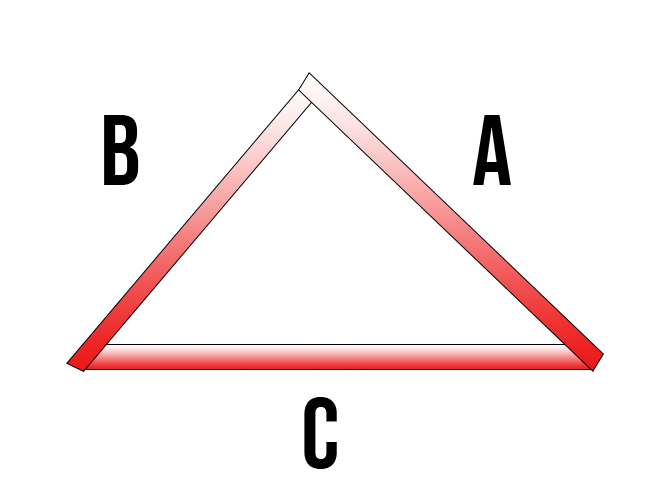a: 5

b: 15

c: 20

If you chose B Congratulations! that is the right answer because applying the theorem the sum of 15+15 is equal to 30 beign higher than 5. Remember that the theorem says that the sum of two sides can't be less or equal to the third side that's why A and C are not correct!

Let's play a zombie game applying today's Lessons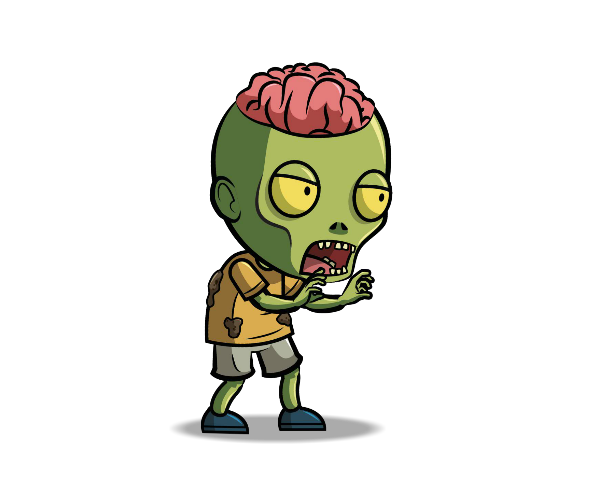A zombie is chasing you on the roof of the flatiron building and there’s an escape to take some secret stairs , which is the quickest way to get therea: Side C

b: Side B

c: I can't escape

If you like this lesson about triangles and you want to learn more in a fun and engaging way go to our Learning Platform Engage!K12  and browse hundreds of lessons for you!